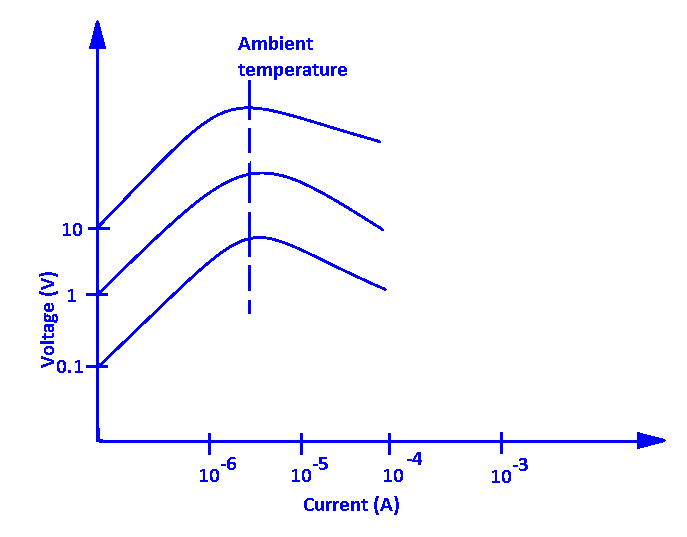# What is Thermistor

A thermistor is also a resistor whose resistance depends on temperature. A thermistor is a temperature-sensitive semiconductor component whose specific resistance changes rapidly with the changing of temperature. in another word, we can say that the resistance of the thermistor is changes when its temperature of its change. A thermistor is also called thermal resistance.

# SymbolA thermistor is a passive component

# Range

• The temperature range at this working is -550C  to +1500C
• The value of resistance a thermistor may have between 0.5Ω to 107Ω

# Types of thermistor

There are 2 types of thermistor

1. NTC
2. PTC

NTC- Negative temperature coefficient – In the NTC thermistor when the temperature increases the resistance decreases. The resistance of the NTC thermistor is inversely proportional to its temperature.

T ∝ RPTC- Positive temperature coefficient– in the PTC when the temperature increases the resistance also increases exponentially, and when temperature decreases resistance decreases.

T ∝ 1/RThermistors are available in different sizes and shapes as per their value and use.

• Disk type
• Rod type
• Probe type
• Washer typeBead type- smallest in size. The diameter of this type of thermistor is 0.015mm to 1.25mm

disk type– Made by processing material under high pressure into cylindrical shapes with diameters of 2.5mm to 25mm.

Probe type– The diameter of this thermistor is 2.5mm and its length varies from 6mm to 50mm.

# Characteristics of thermistors

We will see the characteristics of thermistor only for NTC because of NTC thermistor is widely used

1. resistance-temperature
2. Voltage-current
3. Current-time

## Resistance-temperature characteristics## Voltage-current characteristics

The voltage is going to drop across the thermistor with the increasing the small value of current. As the current increases first the voltage increases, but after the peak value it starts decreasing with the current increasing. The reason behind this is the temperature of the thermistor is not start increasing at some current but after a certain value of current, the temperature of starts increasing in the thermistor and the resistance starts decreasing with the temperature increase. When the resistance decreases the value of voltage drop also decreases.

##Current-time characteristics

Current time characteristics show in the below graph. when we increase the applied voltage then the time delay also increases to reach the maximum current, and when decreasing the voltage level then the time delay to reach the maximum current is also decreasing, this happens because of the heating effect. At the low voltage, the thermistor produces low heat and at lower heat the resistance is high.• Small in size
• high sensitive (especially for NTC)
• fast response and Less expensive

• Not suitable for a wide range of temp (Limited temp. range)
• Needs external DC supply for operation
• Temperature-resistance curve is Non-Linear

# Applications of thermistors

• In household applications like microwave ovens, fridges etc.
• As circuit protection and current limiter
• Temperature sensors
• Self-regulating heating elements and self-regulating overcurrent protectors.
• It is used in vehicles to measure the temperature of coolant and oil.
• Used in a digital thermometer.
• For use to measure the conductivity of electrical components.

A simple circuit using an NTC thermistor is a heat sensor or overheat protection circuit